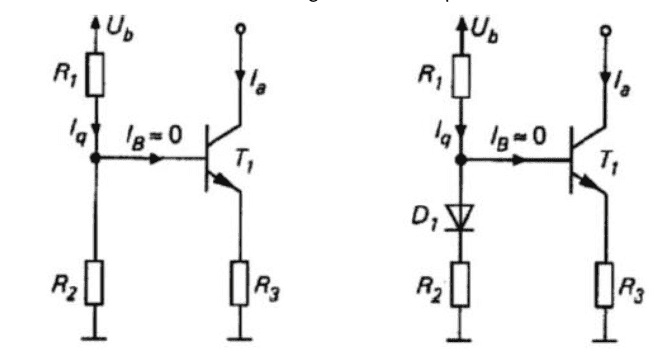# Constant current source transistor circuit - differences between 2 types?

• Engineering
• altruan23
In summary, the statement "Ia = Iq * R2/R3. So Ia is proportional to the ratio of R2/R3." is correct. Vbe is not affected by this equation.f

#### altruan23

Homework Statement
So I am learning about how to create a constant current source with transistors, but I dont understand for what the diode is good for.
Relevant Equations
transistor eq, voltage divider, current divider ruleSo basically we can have a constant current but i don't understand this circuit.
for example: i can have the Ia what ever I want with current divider rule: Ia = Iq * R2/R3. So Ia is proportional to the ratio of R2/R3.
And if i give a resistor at the collector terminal, if i change it between 100-1kohm the Ia is constant however, when I am out of this region, Ia is not constant anymore. why is this ?? and what does the diode on the right circuit help?

Hint -- What effect does temperature have on a bipolar p-n junction? Why is the right circuit better? What is one of the quality measures for a Constant Current Source? (or Constant Current Sink in the case of your two circuits)

Last edited:
•hutchphd, altruan23 and DaveE
I would replace the transistor in the schematic with a simple DC model, then the reason will be more clear.

•altruan23
ok thanks for both answers, I am going to try both tommorow:)

•DaveE and berkeman
I've found that whenever I was confused about a transistor circuit, it's really helpful to just substitute the simplest models (resistors, diodes, current sources, etc.) for the transistor. Then look at the next most complex model and see if you care about the resulting refinement to your answer. Usually the really simple models are enough for you to understand the circuit, more advanced models are to get the details exactly correct. If you are doing this you'll want to separate the DC (low frequency) behavior from the RF (high frequency) behavior. Typically you really only care about one or the other, and the simplified models are quite different.

If you want to see the very most complicated model look up hybrid-π transistor model for AC or ebers-moll for DC. I've never used all of those elements, it's like the whole menu at a restaurant, you end up picking what you care about. IRL, you'll use the really simple versions.

•altruan23
My recommendation: At first, you should try to verify and understand your own statements:
(1) "So basically we can have a constant current"
(2) "Ia = Iq * R2/R3. So Ia is proportional to the ratio of R2/R3."

Is the statement (2) really correct? What about Vbe ?